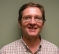## #Matlab rcosfir functionStarted by 6 years ago4 replieslatest reply 6 years ago852 views
figure; plot(rcosfir(.2, 3, 12, 1/9600, 'sqrt') - rcosfir(.2, 3, 12, 1, 'sqrt'))

The difference is negligible. Why does the 'T' parameter (symbol period) seem to have no effect at all? For these two, it's literally equal:

isequal(rcosfir(.2, 3, 12, 1/2400, 'sqrt'), rcosfir(.2, 3, 12, 1/9600, 'sqrt'))

#Matlab

Thanks.

[ - ]Notice that rcosfir generaly computes impulse response:

h(t) = SINC(t/T) COS(pi R t/T)/(1 - 4 R^2 t^2 /T^2)

at time instants t = n*T (where n is integer), which gives us

h(n) = SINC(n) COS(pi R n)/(1 - 4 R^2 n^2)

This, us you noticed, is independent from input signal sampling period.

[ - ]Thank you! Can you explain why they made it an input argument? It seems useless.

[ - ]